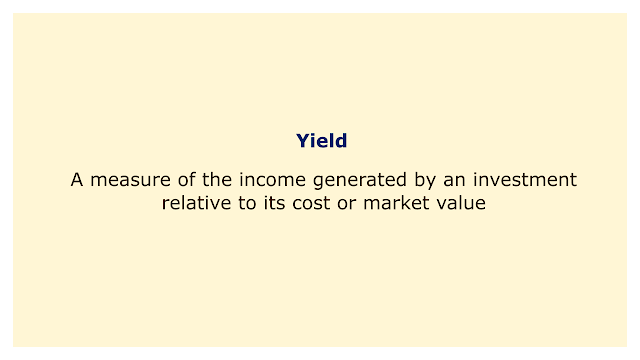# YieldImage: Moneybestpal.com

### Yield is a metric for comparing an investment's income to its cost or market value. A percentage or decimal number is typically used to express it.

The yield, expressed as \$50 divided by \$1,000, is equal to 5% if you purchase a bond for \$1000 that pays \$50 in interest annually. Similarly to this, if you pay \$100 for an equity that pays \$2 in dividends annually, the yield is 2% (\$2/\$100).

Bonds, equities, mutual funds, ETFs, and other types of investments can all be compared using yield. It is crucial to understand that yield is not the same as return. The overall sum of money you make or lose from an investment over time is called a return. Yield is only one component of return, along with capital appreciation or depreciation.

There are different types of yield in finance, depending on how they are calculated and what they represent. Some of the most common ones are:
• Coupon yield: This is the yearly interest rate that a bond pays. The coupon yield, for instance, is 5% on a bond with a face value of \$1,000 and an annual interest payment of \$50. Unless the bond issuer changes the interest rate, the coupon yield does not alter over time.
• Current yield: This is calculated by dividing an investment's annual income by the price at which it is currently trading. The current yield, for instance, is 5.56% (\$50/\$900) for a bond with a face value of \$1000 and an annual interest payment of \$50. As the market price changes over time, the current yield also does.
• Yield to maturity: This represents your overall return if you retain a bond until it matures. It considers the price at the time, the yield on the coupons, and the amount of time to maturity. The yield to maturity, for instance, is 6.38% for a bond with a face value of \$1000, an annual interest payment of \$50, a market price of \$900, and a remaining maturity period of 10 years. Bond ratings are more accurately determined by yield to maturity than by current yield.
• Dividend yield: This is calculated by dividing a stock's annual dividend by its current market price. The dividend yield, for instance, is 2% if a stock's market price is \$100 and its annual dividend payment is \$2. As the market price and dividend amount fluctuate over time, dividend yield also changes.
• Earnings yield: This is calculated by dividing a company's annual profits per share (EPS) by the share price at the current market price. The earnings yield, for instance, is 5% if a company's EPS is \$5 and its market price per share is \$100. Comparing equities with various prices and earnings levels can be done using the earnings yield.

As you can see, yield is an important financial concept that may be used to evaluate the relative worth and income potential of various investments. You should not, however, base all of your financial selections just on this one aspect. In addition, you want to consider variables including risk, liquidity, growth potential, and tax ramifications. Keep in mind that greater returns typically come with higher risks and fewer growth opportunities. As a result, you should constantly maintain a portfolio with investments that are in line with your risk appetite and financial objectives.
Tags